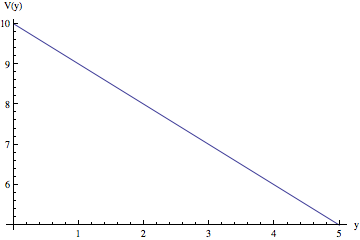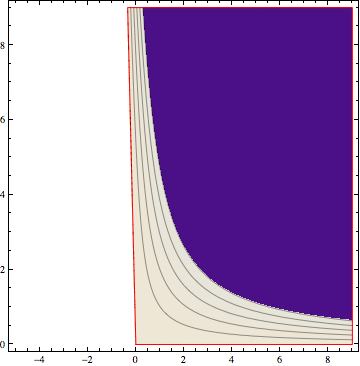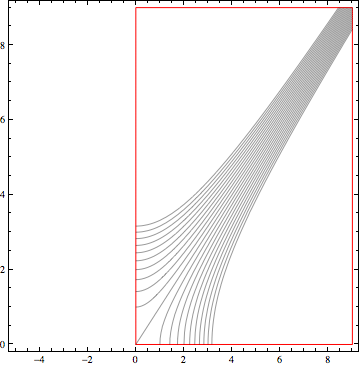# Using Conformal Maps to Find Equipotentials and Field Lines

Note, this relies heavily on the notes from Randy Johnson’s E&M lectures

Let’s start with a uniform electric field in the y direction, of strength $E_0$ and potential $V_0$ at $y=0$. The equation for the potential at any point (y) will then be

Now, we want to use a conformal map to see what the field lines and equipotential lines look like for a similar situation, but with the sides of a plate at an angle $\beta$ to one another.

First, let’s draw the picture of the potential as a function of y:

V[y_] = V0 - E0 y

Plot[V[y] /. {V0 -> 10, E0 -> 1}, {y, 0, 5}, AxesLabel -> {"y", "V(y)"}]Now, we can describe the entire problem in the complex plane, by shifting from $y$ to $z$  f[z_, V0_, E0_, \[Beta]_] := I V0 - E0 z 

And we will use the conformal map from the g plane to the z plane

The inverse is then

and we simply plug this into our $f$ to get the transform.

f[z^(\[Pi]/\[Beta]), v0, e0, \[Beta]]

I V0 - E0 z^(\[Pi]/\[Beta])


The equipotentials are given by the imaginary part of this, and the field lines by the real part. Let’s manipulate the plot to make sure we get physical results.

Be sure to play with the slider!

Im[f[z^(\[Pi]/\[Beta]), v0, e0, \[Beta]]]
-Im[e0 z^(\[Pi]/\[Beta])] + Re[v0]

Re[f[z^(\[Pi]/\[Beta]), v0, e0, \[Beta]]]
-Im[v0] - Re[e0 z^(\[Pi]/\[Beta])]

g1 = Manipulate[
ContourPlot [{-Im[(x + I y)^(\[Pi]/\[Beta])] + Re}, {x, -5,
9}, {y, 0, 9}, AspectRatio -> 1, Contours -> {10, 8, 6, 4, 2, 0},
RegionFunction ->
Function[{x, y}, Im[(x + I y)^(\[Pi]/\[Beta])] >= 0 && y >= 0],
BoundaryStyle -> Red], {\[Beta], \[Pi], 0}]g2 = Manipulate[
ContourPlot [-Im - Re[ (x + I y)^(\[Pi]/\[Beta])], {x, -5,
9}, {y, 0, 9}, AspectRatio -> 1, ContourShading -> False,
Contours -> Range[-10, 10, 1],
RegionFunction ->
Function[{x, y}, Im[(x + I y)^(\[Pi]/\[Beta])] >= 0 && y >= 0],
BoundaryStyle -> Red], {\[Beta], \[Pi], 0}]